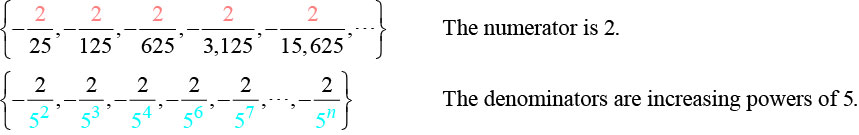# 9.1 Sequences and their notations  (Page 3/15)

 Page 3 / 15

## Writing the terms of an alternating sequence defined by an explicit formula

Write the first five terms of the sequence.

${a}_{n}=\frac{{\left(-1\right)}^{n}{n}^{2}}{n+1}$

Substitute $n=1,$ $n=2,$ and so on in the formula.

$\begin{array}{lll}n=1\hfill & \begin{array}{cc}& \end{array}\hfill & {a}_{1}=\frac{{\left(-1\right)}^{1}{2}^{2}}{1+1}=-\frac{1}{2}\hfill \\ n=2\hfill & \begin{array}{cc}& \end{array}\hfill & {a}_{2}=\frac{{\left(-1\right)}^{2}{2}^{2}}{2+1}=\frac{4}{3}\hfill \\ n=3\hfill & \begin{array}{cc}& \end{array}\hfill & {a}_{3}=\frac{{\left(-1\right)}^{3}{3}^{2}}{3+1}=-\frac{9}{4}\hfill \\ n=4\hfill & \begin{array}{cc}& \end{array}\hfill & {a}_{4}=\frac{{\left(-1\right)}^{4}{4}^{2}}{4+1}=\frac{16}{5}\hfill \\ n=5\hfill & \hfill & {a}_{5}=\frac{{\left(-1\right)}^{5}{5}^{2}}{5+1}=-\frac{25}{6}\hfill \end{array}$

The first five terms are $\left\{-\frac{1}{2},\frac{4}{3},-\frac{9}{4},\frac{16}{5},-\frac{25}{6}\right\}.$

In [link] , does the (–1) to the power of $n$ account for the oscillations of signs?

Yes, the power might be $n,n+1,n-1,\text{\hspace{0.17em}}$ and so on, but any odd powers will result in a negative term, and any even power will result in a positive term.

Write the first five terms of the sequence:

${a}_{n}=\frac{4n}{{\left(-2\right)}^{n}}$

The first five terms are

## Investigating piecewise explicit formulas

We’ve learned that sequences are functions whose domain is over the positive integers. This is true for other types of functions, including some piecewise functions . Recall that a piecewise function is a function defined by multiple subsections. A different formula might represent each individual subsection.

Given an explicit formula for a piecewise function, write the first $\text{\hspace{0.17em}}n\text{\hspace{0.17em}}$ terms of a sequence

1. Identify the formula to which $n=1$ applies.
2. To find the first term, $\text{\hspace{0.17em}}{a}_{1},\text{\hspace{0.17em}}$ use $\text{\hspace{0.17em}}n=1\text{\hspace{0.17em}}$ in the appropriate formula.
3. Identify the formula to which $\text{\hspace{0.17em}}n=2\text{\hspace{0.17em}}$ applies.
4. To find the second term, $\text{\hspace{0.17em}}{a}_{2},\text{\hspace{0.17em}}$ use $\text{\hspace{0.17em}}n=2\text{\hspace{0.17em}}$ in the appropriate formula.
5. Continue in the same manner until you have identified all $\text{\hspace{0.17em}}n\text{\hspace{0.17em}}$ terms.

## Writing the terms of a sequence defined by a piecewise explicit formula

Write the first six terms of the sequence.

Substitute $\text{\hspace{0.17em}}n=1,n=2,\text{\hspace{0.17em}}$ and so on in the appropriate formula. Use ${n}^{2}$ when $n$ is not a multiple of 3. Use $\frac{n}{3}$ when $n$ is a multiple of 3.

The first six terms are

Write the first six terms of the sequence.

The first six terms are

## Finding an explicit formula

Thus far, we have been given the explicit formula and asked to find a number of terms of the sequence. Sometimes, the explicit formula for the $\text{\hspace{0.17em}}n\text{th}\text{\hspace{0.17em}}$ term of a sequence is not given. Instead, we are given several terms from the sequence. When this happens, we can work in reverse to find an explicit formula from the first few terms of a sequence. The key to finding an explicit formula is to look for a pattern in the terms. Keep in mind that the pattern may involve alternating terms, formulas for numerators, formulas for denominators, exponents, or bases.

Given the first few terms of a sequence, find an explicit formula for the sequence.

1. Look for a pattern among the terms.
2. If the terms are fractions, look for a separate pattern among the numerators and denominators.
3. Look for a pattern among the signs of the terms.
4. Write a formula for ${a}_{n}$ in terms of $n.$ Test your formula for and $n=3.$

## Writing an explicit formula for the n Th term of a sequence

Write an explicit formula for the $n\text{th}$ term of each sequence.

1. $\left\{-\frac{2}{11},\frac{3}{13},-\frac{4}{15},\frac{5}{17},-\frac{6}{19},\dots \right\}$
2. $\left\{-\frac{2}{25}\text{,}-\frac{2}{125}\text{,}-\frac{2}{625}\text{,}-\frac{2}{3\text{,}125}\text{,}-\frac{2}{15\text{,}625}\text{,}\dots \right\}$
3. $\left\{{e}^{4}\text{,}{e}^{5}\text{,}{e}^{6}\text{,}{e}^{7}\text{,}{e}^{8}\text{,}\dots \right\}$

Look for the pattern in each sequence.

1. The terms alternate between positive and negative. We can use $\text{\hspace{0.17em}}{\left(-1\right)}^{n}\text{\hspace{0.17em}}$ to make the terms alternate. The numerator can be represented by $n+1.$ The denominator can be represented by $2n+9.$

${a}_{n}=\frac{{\left(-1\right)}^{n}\left(n+1\right)}{2n+9}$

2. The terms are all negative.So we know that the fraction is negative, the numerator is 2, and the denominator can be represented by ${5}^{n+1}.$

${a}_{n}=-\frac{2}{{5}^{n+1}}$
3. The terms are powers of $e.$ For $n=1,$ the first term is ${e}^{4}$ so the exponent must be $n+3.$

${a}_{n}={e}^{n+3}$

how do I set up the problem?
what is a solution set?
Harshika
find the subring of gaussian integers?
Rofiqul
hello, I am happy to help!
Abdullahi
hi mam
Mark
find the value of 2x=32
divide by 2 on each side of the equal sign to solve for x
corri
X=16
Michael
Want to review on complex number 1.What are complex number 2.How to solve complex number problems.
Beyan
yes i wantt to review
Mark
use the y -intercept and slope to sketch the graph of the equation y=6x
how do we prove the quadratic formular
Darius
hello, if you have a question about Algebra 2. I may be able to help. I am an Algebra 2 Teacher
thank you help me with how to prove the quadratic equation
Seidu
may God blessed u for that. Please I want u to help me in sets.
Opoku
what is math number
4
Trista
x-2y+3z=-3 2x-y+z=7 -x+3y-z=6
can you teacch how to solve that🙏
Mark
Solve for the first variable in one of the equations, then substitute the result into the other equation. Point For: (6111,4111,−411)(6111,4111,-411) Equation Form: x=6111,y=4111,z=−411x=6111,y=4111,z=-411
Brenna
(61/11,41/11,−4/11)
Brenna
x=61/11 y=41/11 z=−4/11 x=61/11 y=41/11 z=-4/11
Brenna
Need help solving this problem (2/7)^-2
x+2y-z=7
Sidiki
what is the coefficient of -4×
-1
Shedrak
the operation * is x * y =x + y/ 1+(x × y) show if the operation is commutative if x × y is not equal to -1
An investment account was opened with an initial deposit of \$9,600 and earns 7.4% interest, compounded continuously. How much will the account be worth after 15 years?
lim x to infinity e^1-e^-1/log(1+x)
given eccentricity and a point find the equiationByByBy Rohini AjayBy Nicole DuquetteBy OpenStaxBy Stephen VoronBy Stephen VoronBy John GabrieliBy Jazzycazz JacksonBy Brooke DelaneyBy Heather McAvoyBy Brooke Delaney